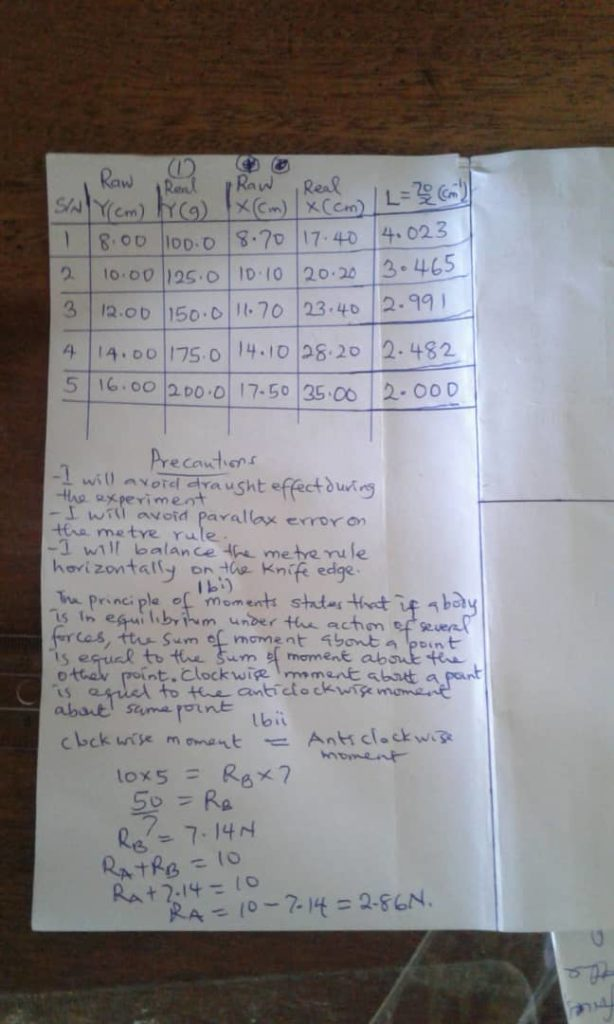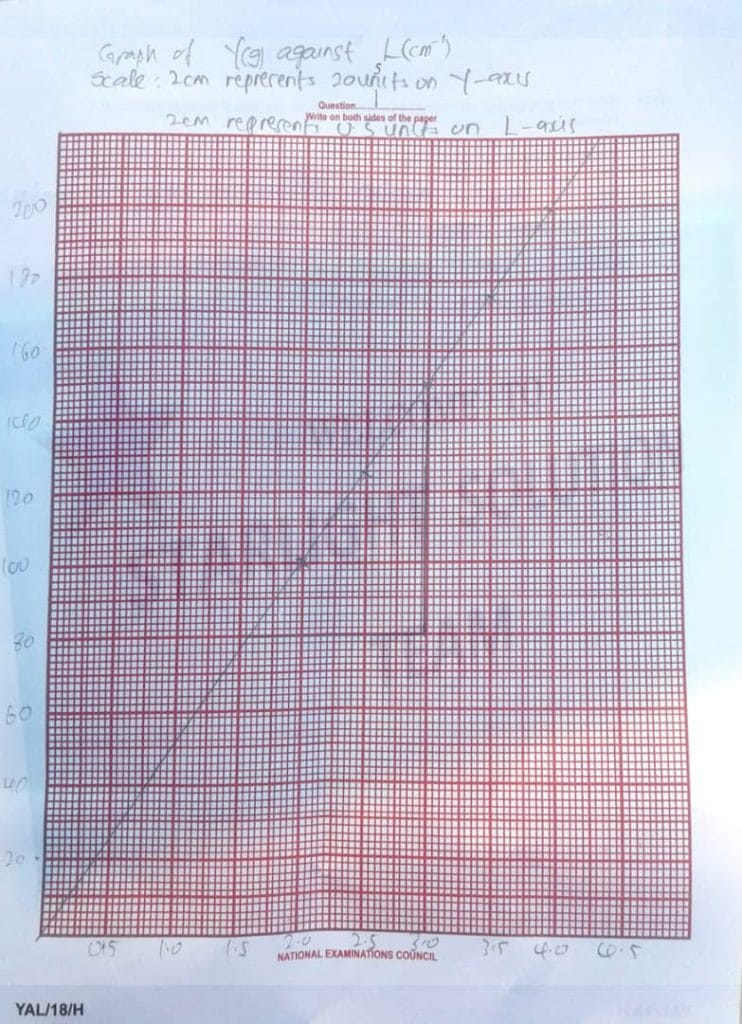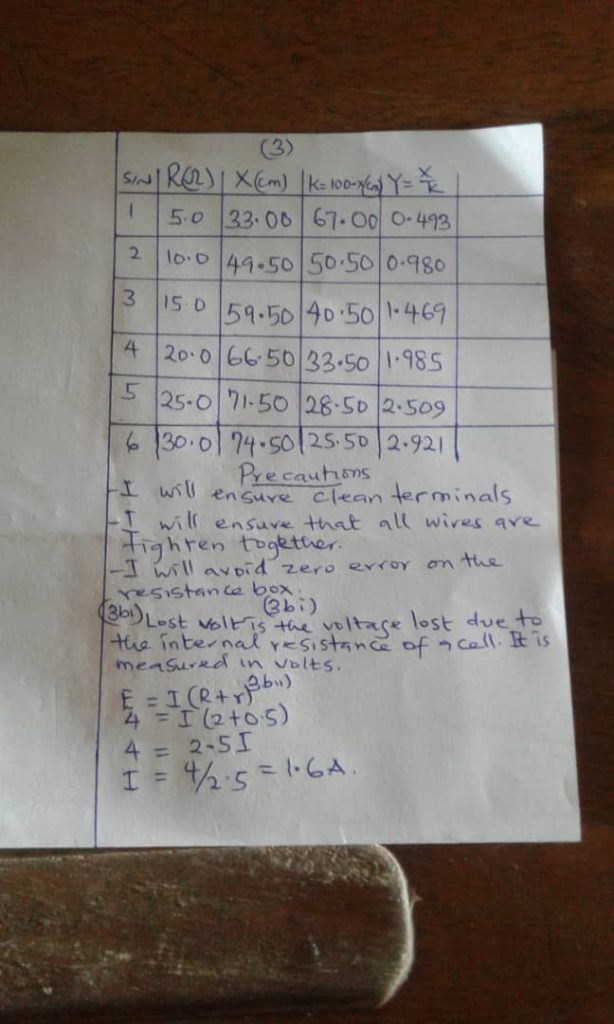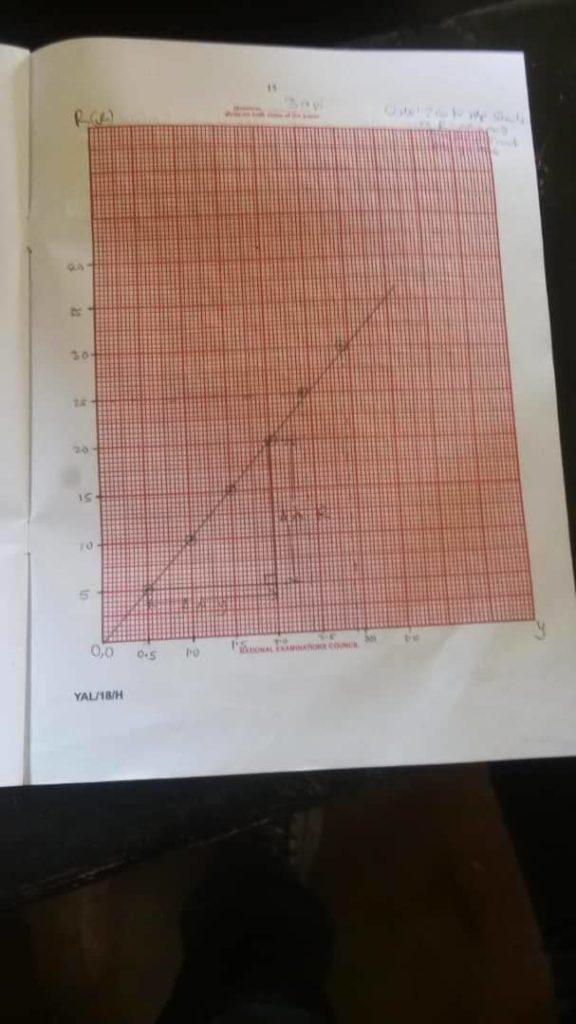# NECO GCE Physics Practical Questions And Answers For 2018=-=-=-=–=-=-=-=–=-=-=-=–=-=-=-=-=-=-=-=–=-=-=-=–=-=-=-=–=-=-=-=-

No 1)

(1aiv)
In a tabular form
Under S/N
1.0, 2.0, 3.0, 4.0, 5.0

Under y(g)
8.00, 10.00, 12.00, 14.00, 16.00

[Under yR(g)= 12.5*y]
100.00, 125.00, 150.00, 175.00, 200.00

Under X(cm)
17.60, 14.00, 11.60, 10.00, 8.70

Under XR(cm) = x * 2
35.00, 28.00, 23.20, 20.00, 17.40

Under L = 70/X
2.000, 2.500, 3.000, 3.500, 4.00

DRAW ANOTHER TABLE OF y(g) and L(cm)
Under y(g)
100.00
125.00
150.00
175.00
200.00

Under L(cm)
2.00
2.50
3.00
3.50
4.00

(1av)
DRAW THE GRAPH

(1avi)
Slope(s) = Dy/DL =
y2 – y1/L2 – L1
Where y2 = 175g, y1 = 100g
L2 = 3.5cm, L1 = 2.00cm

Slope(s) = 175 – 100/3.5 – 2

(1avii)
(PICK TWO)
(i)I ensured mass did not touch/rest on the table
(ii)I avoided error due to parallax on metre rule
(iii)I noted zero error on metre rule
(iv)I avoided draught

(1bi)
The principal of moments state that if a body is in equilibrium,then the sum of the clockwise moments about any point on the body is equal to the sum of the anticlockwise moments about the same point

(1bii)
Draw the diagram

Taking moments about point B ie CWM = ACM
(FA7) = 102
7F = 20
7F/7 = 20/7
F = 2.9N
= 75/1.5
= 50g/cm

(b)
-i- The Principle of Moments states that when in equilibrium the total sum of the anti-clockwise moment is equal to the total sum of the clockwise moment. When a system is stable or balance it is said to be in equilibrium as all the forces acting on the system cancel each other out.-=-=-=-=–=-=-=-=–=-=-=-=–=-=-=-=-=-=-=-=–=-=-=-=–=-=-=-=–=-=-=-=-
No 3)

(a)
-viii-
• I would have ensured that the electrical wires were tightly connected to the terminals in order to avoid current fluctuations.
• I would have ensured that the key is removed whenever the electric circuit is not in use so as not to allow the battery to run down.

(3a)
In a tabular form
Under S/N
1, 2, 3, 4, 5, 6

Under R(ohms)
5.0, 10.0, 15.0, 20.0, 25.0, 30.0

Under X(cm)
33.00, 49.50, 60.00, 67.00, 71.00, 74.50

Under K = 100 -X(cm)
67.00, 50.50, 40.00, 33.00, 29.00, 25.50

Under Y = x/k
0.4925, 0.9802, 1.5000, 2.0303, 2.4483, 2.9216

(3avi)
DRAW THE GRAPH

(3avii)
Slope
R2 – R1/y2 -y1
= 20 – 5/2.0 – 0.5
= 15/1.5
=10.0 ohms

(3aviii)
(i) I must use a freshly charged accumulator.
(ii) I must take proper readings through my resistance box and potentiometer to avoid error due to parallax

(3bi)
Lost volt is the voltage lost due to the internal resistance of a cell. It is measured in volts.

(3bii)
E = I(R+r)
4 = I(2+0.5)
4 = 2.5I
I = 4/2.5
= 1.6A!!!!!

-=-=-=-=–=-=-=completed-=–=-=-=-=-=-=-=-=–=-=-=-=–=-=-=-=–=-=-=-=-

NECO GCE Physics Practical Questions And Answers For 2018